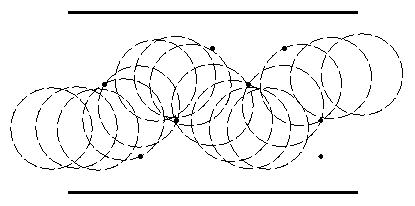Not an official ACM page
[Problem E | 1994 Western European Regional problem set | My ACM problem archive | my home page]

# Problem E

## Fatman

Some of us may be so fortunate to be thin enough to squeeze through the tiniest hole, others are not. Getting from A to B in a crowded supermarket (even without a cart) can be tough and may require sophisticated navigation: there may seem to be enough room on the one side, but then you may run into trouble with that lady further down...

Let's consider this in an abstract fashion: given an aisle of a certain width, with infinitely small obstacles scattered around, just how fat can a person be and still be able to get from the left side to the right side. Assume that seen from above a (fat) person looks like a circle and the person is incompressible (a person with diameter d cannot go between two obstacles having distance less than d).

### Input

The first line of input specifies the number of test cases your program has to process. The input for each test case consists of the following lines:
• One line with the integer length L (0 <= L <= 100) and integer width W (0 <= W <= 100) of the aisle, separated by a single space.
• One line with the number of obstacles N (0 <= N <= 100) in the aisle.
• N lines, one for each obstacle, with its integer coordinates X and Y (0 <= X <= L, 0 <= Y <= W) separated by a single space.

### Output

For each test case given in the input, print a line saying '`Maximum size in test case N is M.`', where M is rounded to the nearest fractional part of exactly four digits. M is the maximum diameter of a person that can get through the aisle specified for that test case. N is the current test case number, starting at one.

```1
8 5
8
2 1
1 3
3 2
4 4
5 3
6 4
7 2
7 1
```

### Sample Output

```Maximum size in test case 1 is 2.2361.
```
The sample input looks like: